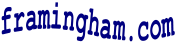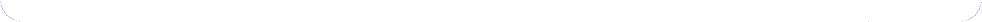# Kids: Homework Help: Math

## I Hate Math!

"I don't get it!" "I'll never need this stuff!" "When I grow up, I'm not going to become a (fill in blank) so I won't need math!" "I hate math!!!"

The truth is, math is all around. You use math to play games, and to keep score. You use math to tell time, to count money, to bake a cake, to find a radio or t.v. station, and lots of other activities where numbers are involved.

"Fractions are stupid!" - fractions aren't as easy as counting "1, 2, 3..", but chances are you'll need to know them later on.

Temperature Converter - need to convert Farenheit and Celsius temperatures?   Handy only converter, formula and links about temperature

Definition of Fractions
 1/2 = one of two equal parts 1/4 = one of four equal parts 1/8 = one of eight equal parts 3/8 = three of eight equal parts 1/10 = one of ten equal parts
Fraction = Decimal
 1/2 = .5 1/4 = .25 1/8 = .125 1/3 = .333... 3/4 = .75 7/8 = .875 1/10 = .1 1/100 = .01 1/1000 = .001
Inches, Feet & Yards
 1 foot = 12 inches 1 yard = 3 feet 1 yard = 36 inches

### More Math Links

The Math Forum - K-12, College, and Advanced Math Resources.   And if you can't find the answer to a problem.... Ask Dr. Math!

Math Glossary - illustrated and animated site explains math terms for grades 1 to 8.